# Week 5 - Chemistry Flashcards

Set Details Share
created 3 years ago by SkyRose
300 views
Subjects:
chemistry
Page to share:
Embed this setcancel
COPY
code changes based on your size selection
Size:
X

1

In a covalent bond

A) two atoms exchange electrons and the ions are attracted to one another.

B) two ions come together and form a crystal lattice.

C) two atoms share valence electrons and those shared electrons form the chemical bond.

D) two elements react and form a new compound.

C) two atoms share valence electrons and those shared electrons form the chemical bond.

2

Which of the following is an example of a covalent bond?

A) K - Cl

B) Al - Cl

C) H - Cl

D) Al - Al

C) H - Cl

3

What is the correct IUPAC name for BrCl₃?

bromine trichloride

4

What is the correct IUPAC name for CBr₄?

carbon tetrabromide

5

What is the correct IUPAC name for CBr₄?

carbon tetrabromide

6

What is the correct IUPAC name for NI₃?

nitrogen triiodide

7

What is the correct IUPAC name for N₂O₄?

dinitrogen tetroxide

8

What is the correct IUPAC name for P₄S₃?

tetraphosphorus trisulfide

9

What is the correct IUPAC name for SeO₂?

selenium dioxide

10

What is the correct IUPAC name for S₂Cl₈?

disulfur octachloride

11

What is the correct IUPAC name for TeBr₆?

tellurium hexabromide

12

What is the correct IUPAC name for Se₂I₈?

diselenium octaiodide

13

What is the correct IUPAC name for SO₃?

sulfur trioxide

14

What is the correct IUPAC name for TeF₄?

tellurium tetrafluoride

15

What is the correct IUPAC name for AsBr₃?

arsenic tribromide

16

What is the correct IUPAC name for As₂S₅?

diarsenic pentasulfide

17

What is the correct IUPAC name for SbCl₃?

antimony trichloride

18

Write the chemical formula for arsenic pentafluoride

AsF₅

19

Write the chemical formula for bromine pentachloride

BrCl₅

20

Write the chemical formula for carbon tetrafluoride

CF₄

21

Write the chemical formula for dichlorine heptoxide

Cl₂O₇

22

Write the chemical formula for dinitrogen tetroxide

N₂O₄

23

Write the chemical formula for diphosphorus pentabromide

P₂Br₅

24

Write the chemical formula for nitrogen trichloride

NCl₃

25

Write the chemical formula for selenium hexafluoride

SeF₆

26

Write the chemical formula for sulfur tetraiodide

SI₄

27

Write the chemical formula for tellurium hexaiodide

TeI₆

28

Write the chemical formula for tetraphosphorus hexasulfide

P₄S₆

P₄S₆

29

Which has the correct name-formula combination?

A) Chlorine pentafluoride - ClF

B) Diphosphorus pentoxide - P₂O

C) Carbon tetraiodide - CI₄

D) Sulfur tetrafluoride - SF

C) Carbon tetraiodide - CI₄

30

Which has the incorrect name-formula combination?

A) Sulfur dioxide - SO₂

B) Iodine trifluoride - IF

C) Nitrogen tribromide - NBr₃

D) Silicon tetrafluoride - SiF₄

B) Iodine trifluoride - IF

31

Which of the following is the correct Lewis symbol for a neutral atom of Al?

A) see problem image

B) see problem image

C) see problem image

D) see problem image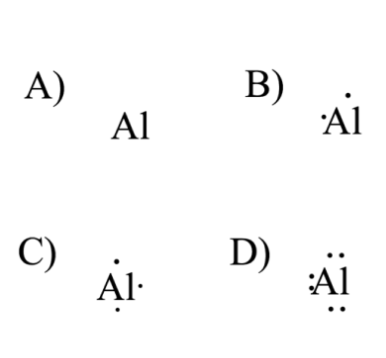C) see problem image

32

Which of the following is the correct Lewis symbol for a neutral atom of F?

A) see problem image

B) see problem image

C) see problem image

D) see problem image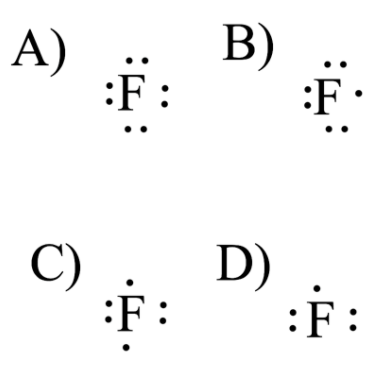B) see problem image

33

Which of the following is the correct Lewis symbol for a neutral atom of O?

A) see problem image

B) see problem image

C) see problem image

D) see problem image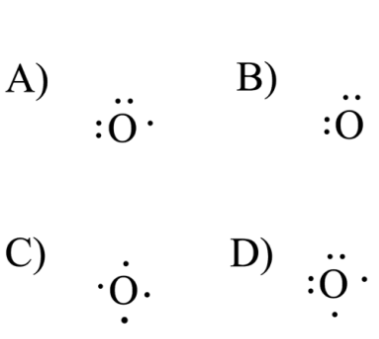D) see problem image

34

Which of the following is not a valid Lewis symbol?

A) see problem image

B) see problem image

C) see problem image

D) see problem image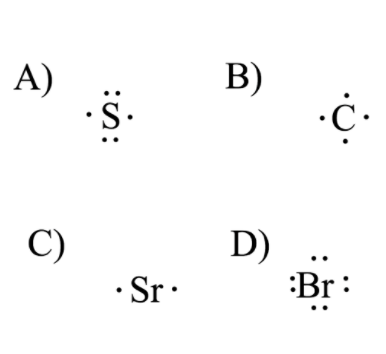D) see problem image

35

Draw the Lewis structure of ammonia (NH₃).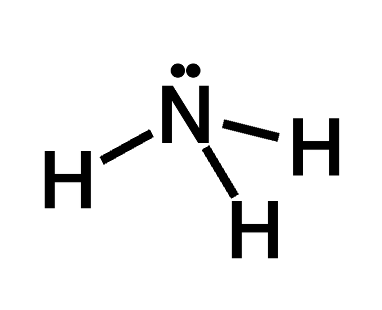36

Draw the Lewis structure of carbon dioxide (CO₂).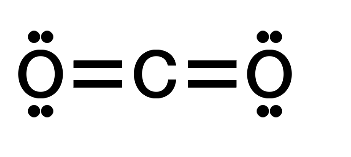37

Draw the Lewis structure of Cl₂.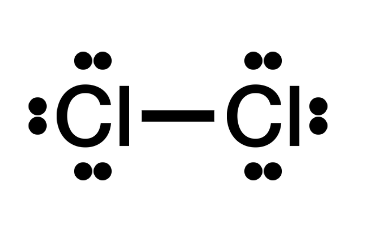38

Draw the Lewis structure of formaldehyde (H₂CO).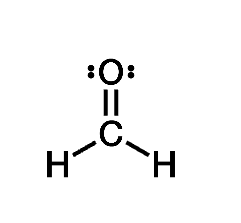39

Draw the Lewis structure of H₂.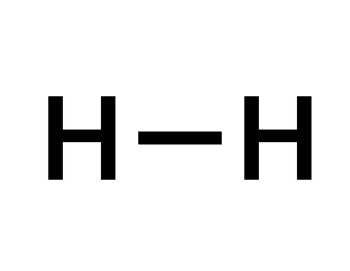40

Draw the Lewis structure of N₂.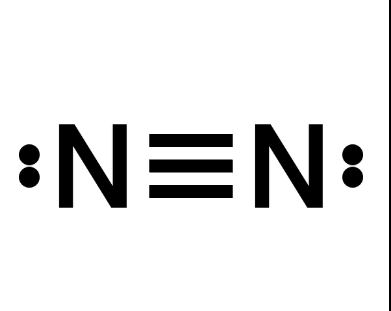41

Draw the Lewis structure of O₂.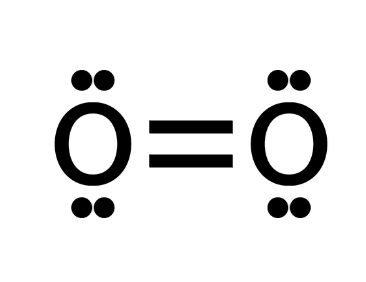42

Draw the Lewis structure of water (H₂O).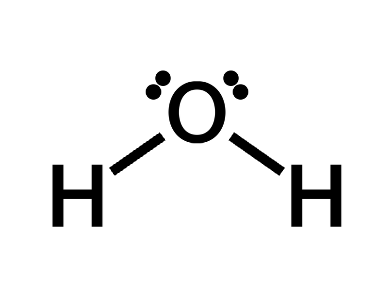43

A skeletal structure for SO₂Cl₂ is shown below. Starting from this structure, complete the Lewis structure that follows the octet rule on all atoms.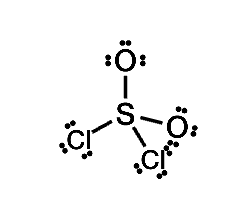44

Determine the number of valence electrons in HCN and then draw the corresponding Lewis structure.

A) 13

B) 12

C) 10

D) 9

E) 7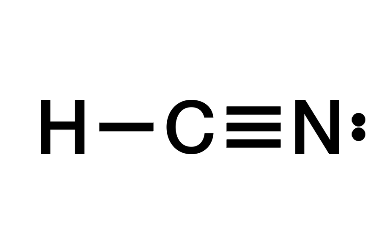C) 10

45

Determine the number of valence electrons in POCl₃ and then draw the corresponding Lewis structure (by following the octet rule on all atoms).

A) 30

B) 32

C) 28

D) 31

E) 35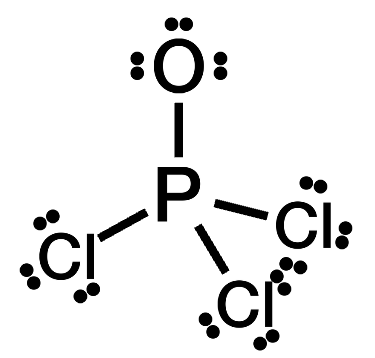B) 32

46

The Lewis structure for NI₃ shown below is incorrect. Starting from this structure, complete the correct structure.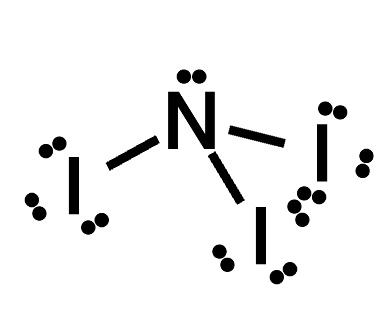47

The Lewis structure for SiS₂ shown below is incorrect. Starting from this structure, complete the correct structure.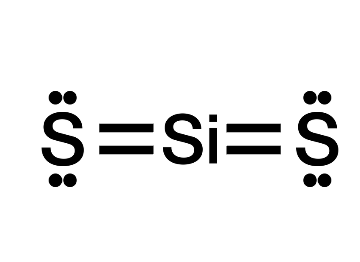48

Determine the number of valence electrons in ethene (C₂H₄) and then draw the corresponding Lewis structure.

A) 11

B) 13

C) 12

D) 15

E) 14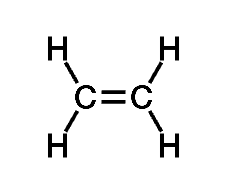C) 12

49

The Lewis structure for ethyne (C₂H₂) shown below is incorrect. Starting from this structure, complete the correct structure.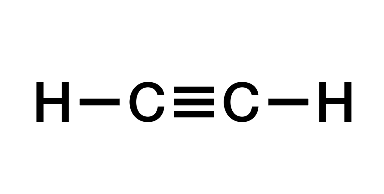50

Determine the number of valence electrons in nitrous acid (HNO₂, sometimes written as HONO) and then draw the corresponding Lewis structure.

A) 22

B) 18

C) 19

D) 15

E) 17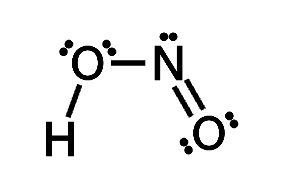B) 18

51

A single bond, compared to a double bond,

A) is longer.

B) is stronger.

C) has a higher bond dissociation energy.

D) is not capable of rotation.

A) is longer.

52

Which of the following has the shortest bond length?

A) a single bond

B) a double bond

C) a triple bond

C) a triple bond

53

Which of the following has the shortest bond length?

A) a single bond

B) a double bond

C) a triple bond

C) a triple bond

54

Which one of the following statements best describes electronegativity in atoms?

A) Electronegativity is what happens when an atom gains an electron to become an anion.

B) Electronegativity is the attraction an element's nucleus has for the electrons in a chemical bond.

C) Electronegativity is the energy lost when an atom gains an electron.

D) Electronegativity is the energy absorbed when an atom loses an electron.

B) Electronegativity is the attraction an element's nucleus has for the electrons in a chemical bond.

55

Which one of the following is the least electronegative atom?

A) Na

B) Cl

C) P

D) Al

A) Na

56

Which group in the periodic table tends to have high electronegativity values?

A) the alkali metals

B) the noble gases

C) the halogens

D) the transition metals

C) the halogens

57

Which of the following bonds is a nonpolar covalent bond?

A) H - F

B) N - F

C) F - Cl

D) F - F

D) F - F

58

Which of the following bonds is a polar covalent bond?

A) Li - Cl

B) Cl - Cl

C) H - Cl

D) Li - Li

C) H - Cl

59

Based on their positions in the periodic table, which of the following bonds is the most polar?

A) O - F

B) C - F

C) B - F

D) F - F

C) B - F

60

In a polar covalent bond,

A) both atoms in the bond have the same level of electronegativity.

B) one atom in the bond must have higher electronegativity than the other atom.

C) one atom must be a H atom.

D) both atoms in the bond must be metals.

B) one atom in the bond must have higher electronegativity than the other atom.

61

Which one of the following statements is FALSE?

A) Ionic bonding results from the transfer of electrons from one atom to another

B) Dipole moments result from the unequal distribution of electrons in a molecule

C) The electrons in a polar bond are found nearer to the more electronegative element

D) A molecule with very polar bonds can be nonpolar

E) Linear molecules cannot have a net dipole moment

E) Linear molecules cannot have a net dipole moment

62

What is the correct molecular geometry of NH₃ according to Valence Shell Electron Pair Repulsion Theory?

A) trigonal planar

B) trigonal pyramidal (pyramidal)

C) bent

D) tetrahedral

B) trigonal pyramidal (pyramidal)

63

Draw the Lewis structure of chloroform (CHCl₃) and then determine the ideal bonding angle(s) of the central atom.

A) 180º

B) 90º and 120º

C) 109.5º

D) 120º

E) 135º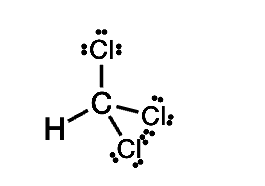C) 109.5º

64

Draw the Lewis structure of CHOCl and then determine the ideal bonding angle(s) of the central atom.

A) 120º

B) 45º

C) 135º

D) 90º

E) 60º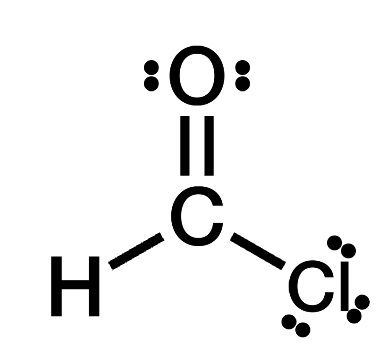A) 120º

65

Draw the Lewis structure of ClF₃ (with minimized formal charges) and then determine its electron domain and molecular geometries.

A) trigonal bipyramidal / pentagonal

B) trigonal bipyramidal / t-shaped

C) trigonal / pyramidal

D) trigonal / square planar

E) pyramidal / pentagonal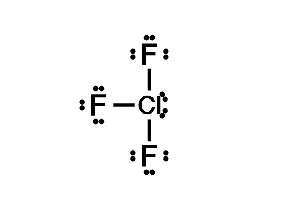B) trigonal bipyramidal / t-shaped

66

Draw the Lewis structure of ethyne (C₂H₂) and then choose the appropriate pair of molecular geometries of the two central atoms. Your answer choice is independent of the orientation of your drawn structure.

A) linear / linear

B) trigonal / pyramidal

C) pyramidal / trigonal

D) trigonal pyramidal / trigonal pyramidal

E) planar / linear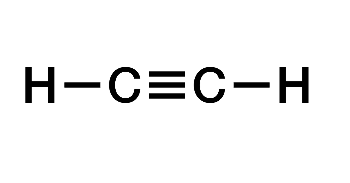A) linear / linear

67

Draw the Lewis structure of H₂CS and then determine its electron domain and molecular geometries.

A) planar / tetrahedral

B) trigonal planar / linear

C) trigonal / trigonal planar

D) trigonal planar / trigonal planar

E) planar / planar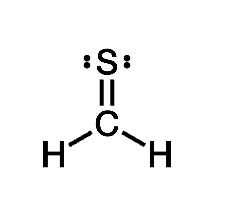D) trigonal planar / trigonal planar

68

Draw the Lewis structure of methanol (CH₃OH) and then choose the appropriate pair of molecular geometries of the two central atoms. Your answer choice is independent of the orientation of your drawn structure.

A) bent (109.5º) / bent (120º)

B) tetrahedral / bent (109.5º)

C) trigonal pyramidal / planar

D) planar / trigonal

E) bent (120º) / planar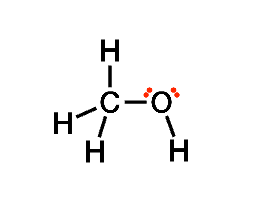B) tetrahedral / bent (109.5º)

69

Draw the Lewis structure of ozone (O₃) and then determine its electron domain and molecular geometries.

A) planar / trigonal planar

B) trigonal planar / bent (120º)

C) trigonal / trigonal planar

D) planar / trigonal

E) pyramidal / pyramidal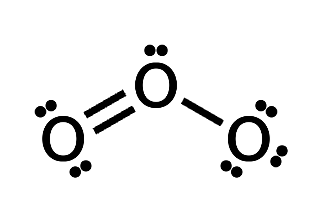B) trigonal planar / bent (120º)

70

Draw the Lewis structure of PH₃ and then determine its electron domain and molecular geometries.

A) tetrahedral / bent (109.5º)

B) tetrahedral / trigonal pyramidal

C) trigonal / tetrahedral

D) planar / trigonal pyramidal

E) trigonal planar / trigonal planarB) tetrahedral / trigonal pyramidal

71

Draw the Lewis structure of silicon dioxide (SiO₂) and then determine its electron domain and molecular geometries.

A) planar / trigonal pyramidal

B) trigonal planar / bent (120º)

C) planar / trigonal

D) linear / pyramidal

E) linear / linear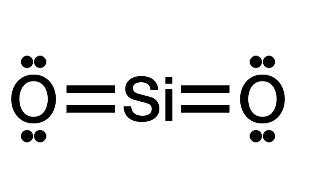E) linear / linear

72

Draw the Lewis structure of water (H₂O) and then determine its electron domain and molecular geometries.

A) trigonal planar / trigonal

B) trigonal planar / planar

C) tetrahedral / bent (109.5º)

D) tetrahedral / linear

E) tetrahedral / trigonal planar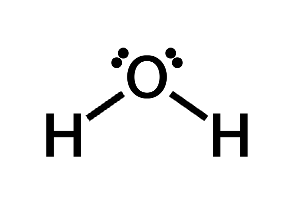C) tetrahedral / bent (109.5º)

73

Which of the following bonds is the most polar?

A) P - S

B) C - Cl

C) F - F

D) Rb - Br

E) All bonds have equal polarity.

D) Rb - Br

74

Draw the Lewis structure of carbon dioxide (CO₂) and then determine if the molecule is polar or nonpolar.

A) Nonpolar

B) Polar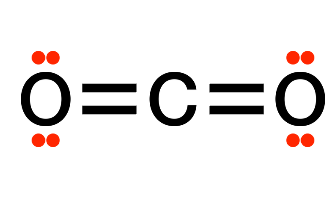A) Nonpolar

75

Draw the Lewis structure of carbon tetrafluoride (CF₄) and then determine if the molecule is polar or nonpolar.

A) Nonpolar

B) Polar+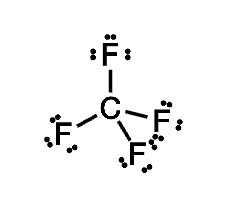A) Nonpolar

76

Draw the Lewis structure of formaldehyde (H₂CO) and then determine if the molecule is polar or nonpolar.

A) Nonpolar

B) Polar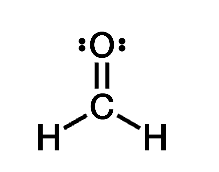B) Polar

77

Draw the Lewis structure of O₂ and then determine if the molecule is polar or nonpolar.

A) Nonpolar

B) Polar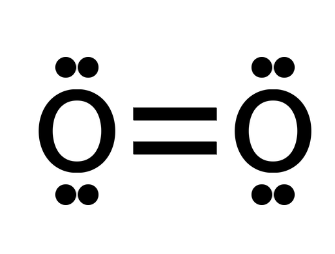A) Nonpolar

78

Draw the Lewis structure of water (H₂O) and then determine if the molecule is polar or nonpolar.

A) Nonpolar

B) Polar+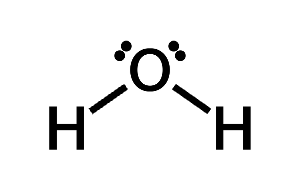B) Polar

79

Draw the Lewis structure of PBr₃ and then determine if the molecule is polar or nonpolar.

A) Nonpolar

B) Polar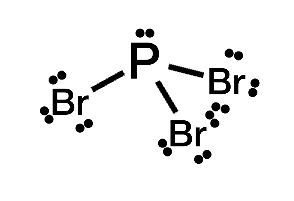B) Polar

80

How would you determine the formula weight of NaCl?

A) Add the mass of sodium (22.99 amu) plus the mass of chlorine together (35.45 amu).

B) Multiply the mass of sodium (22.99 amu) by the mass of chlorine (35.45 amu).

C) Divide the mass of chlorine (35.45 amu) by the mass by sodium (22.99 amu).

D) Subtract the mass of chlorine (35.45 amu) from the mass of sodium (22.99 amu).

A) Add the mass of sodium (22.99 amu) plus the mass of chlorine together (35.45 amu).

81

What are the similarities in how formula weight and molar mass are calculated?

A) Formula weight and molar mass are both calculated by taking the individual atoms' masses in the formula, multiplying them by how many there are in the formula, and then adding the numbers together.

B) Formula weight and molar mass are both calculated by taking the individual atoms' masses in the formula, multiplying them by how many there are in the formula, and then subtracting the numbers from each other.

C) Formula weight and molar mass are both calculated by taking the individual atoms' masses in the formula, dividing them by how many there are in the formula, and then adding the numbers together.

D) Formula weight and molar mass are both calculated by taking the individual atoms mass in the formula, dividing them by how many there are in the formula, and then subtracting the numbers from each other.

A) Formula weight and molar mass are both calculated by taking the individual atoms' masses in the formula, multiplying them by how many there are in the formula, and then adding the numbers together.

82

Determine the formula weight of KBr.

119.00 amu

83

Determine the molar mass of CH₃OCH₃.

46.08 g/mol

84

Determine the molar mass of CH₃OH.

32.05 g/mol

85

Determine the molar mass of C₆H₁₂O₆.

180.18 g/mol

86

Which one of the following has the smallest molar mass?

A) Ca(NO₃)₂

B) Ca₃N₂

C) Ca(C₂H₃O₂)₂

D) CaSO₄

D) CaSO₄

87

The number of covalent bonds carbon forms in organic compounds is ____.

A) 2

B) 3

C) 4

D) 5

E) 6

C) 4

88

Which of the following formulas represents an organic compound?

A) KCl

B) NH₃

C) Cl₂

D) C₃H₈

E) SiH₄

D) C₃H₈

89

A hydrocarbon is referred to as __________ if all the bonds in the molecule are single bonds.

A) aromatic

B) saturated

C) unsaturated

D) planar

E) octahedral

B) saturated